The National Council of Educational Research and Training (NCERT) is an autonomous body of the Indian government that formulates the curricula for schools in India that are governed by the Central Board of Secondary Education (CBSE) and certain state boards. Therefore, students who will be taking the Class 10 tests administered by various boards should consult this NCERT Syllabus in order to prepare for those examinations, which in turn will assist those students get a passing score.

When working through the exercises in the NCERT textbook, if you run into any type of difficulty or uncertainty, you may use the swc NCERT Solutions for class 9 as a point of reference. While you are reading the theory form textbook, it is imperative that you always have notes prepared. You should make an effort to understand things from the very beginning so that you may create a solid foundation in the topic. Use the NCERT as your parent book to ensure that you have a strong foundation. After you have finished reading the theoretical section of the textbook, you should go to additional reference books.

### NCERT Solutions for Class 9 Maths Exercise 8.1

Question 1. The angles of quadrilateral are in the ratio 3 : 5 : 9 : 13. Find all the angles of the quadrilateral.
Solution:
Let the angles of the quadrilateral be 3x, 5x, 9x and 13x.
∴ 3x + 5x + 9x + 13x = 360°
[Angle sum property of a quadrilateral]
⇒ 30x = 360°
⇒ x =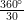= 12°
∴ 3x = 3 x 12° = 36°
5x = 5 x 12° = 60°
9x = 9 x 12° = 108°
13a = 13 x 12° = 156°
⇒ The required angles of the quadrilateral are 36°, 60°, 108° and 156°.

Question 2. If the diagonals of a parallelogram are equal, then show that it is a rectangle.
Solution:
Let ABCD is a parallelogram such that AC = BD.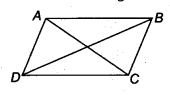In ∆ABC and ∆DCB,
AC = DB [Given]
AB = DC [Opposite sides of a parallelogram]
BC = CB [Common]
∴ ∆ABC ≅ ∆DCB [By SSS congruency]
⇒ ∠ABC = ∠DCB [By C.P.C.T.] …(1)
Now, AB || DC and BC is a transversal. [ ∵ ABCD is a parallelogram]
∴ ∠ABC + ∠DCB = 180° … (2) [Co-interior angles]
From (1) and (2), we have
∠ABC = ∠DCB = 90°
i.e., ABCD is a parallelogram having an angle equal to 90°.
∴ ABCD is a rectangle.

Question 3. Show that if the diagonals of a quadrilateral bisect each other at right angles, then it is a rhombus.
Solution:
Let ABCD be a quadrilateral such that the diagonals AC and BD bisect each other at right angles at O.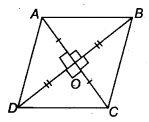∴ In ∆AOB and ∆AOD, we have
AO = AO [Common]
OB = OD [O is the mid-point of BD]
∠AOB = ∠AOD [Each 90]
∴ ∆AQB ≅ ∆AOD [By,SAS congruency
∴ AB = AD [By C.P.C.T.] ……..(1)
Similarly, AB = BC .. .(2)
BC = CD …..(3)
CD = DA ……(4)
∴ From (1), (2), (3) and (4), we have
AB = BC = CD = DA
Thus, the quadrilateral ABCD is a rhombus.
Alternatively : ABCD can be proved first a parallelogram then proving one pair of adjacent sides equal will result in rhombus.

Question 4. Show that the diagonals of a square are equal and bisect each other at right angles.
Solution:
Let ABCD be a square such that its diagonals AC and BD intersect at O.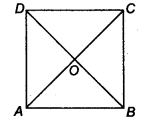(i) To prove that the diagonals are equal, we need to prove AC = BD.
In ∆ABC and ∆BAD, we have
AB = BA [Common]
BC = AD [Sides of a square ABCD]
∠ABC = ∠BAD [Each angle is 90°]
∴ ∆ABC ≅ ∆BAD [By SAS congruency]
AC = BD [By C.P.C.T.] …(1)

(ii) AD || BC and AC is a transversal. [∵ A square is a parallelogram]
∴ ∠1 = ∠3
[Alternate interior angles are equal]
Similarly, ∠2 = ∠4
Now, in ∆OAD and ∆OCB, we have
AD = CB [Sides of a square ABCD]
∠1 = ∠3 [Proved]
∠2 = ∠4 [Proved]
∴ ∆OAD ≅ ∆OCB [By ASA congruency]
⇒ OA = OC and OD = OB [By C.P.C.T.]
i.e., the diagonals AC and BD bisect each other at O. …….(2)

(iii) In ∆OBA and ∆ODA, we have
OB = OD [Proved]
BA = DA [Sides of a square ABCD]
OA = OA [Common]
∴ ∆OBA ≅ ∆ODA [By SSS congruency]
⇒ ∠AOB = ∠AOD [By C.P.C.T.] …(3)
∵ ∠AOB and ∠AOD form a linear pair.
∴∠AOB + ∠AOD = 180°
∴∠AOB = ∠AOD = 90° [By(3)]
⇒ AC ⊥ BD …(4)
From (1), (2) and (4), we get AC and BD are equal and bisect each other at right angles.

Question 5. Show that if the diagonals of a quadrilateral are equal and bisect each other at right angles, then it is a square.
Solution:
Let ABCD be a quadrilateral such that diagonals AC and BD are equal and bisect each other at right angles.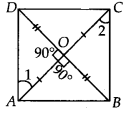Now, in ∆AOD and ∆AOB, We have
∠AOD = ∠AOB [Each 90°]
AO = AO [Common]
OD = OB [ ∵ O is the midpoint of BD]
∴ ∆AOD ≅ ∆AOB [By SAS congruency]
⇒ AD = AB [By C.P.C.T.] …(1)
Similarly, we have
AB = BC … (2)
BC = CD …(3)
CD = DA …(4)
From (1), (2), (3) and (4), we have
AB = BC = CD = DA
∴ Quadrilateral ABCD have all sides equal.
In ∆AOD and ∆COB, we have
AO = CO [Given]
OD = OB [Given]
∠AOD = ∠COB [Vertically opposite angles]
So, ∆AOD ≅ ∆COB [By SAS congruency]
∴∠1 = ∠2 [By C.P.C.T.]
But, they form a pair of alternate interior angles.
Similarly, AB || DC
∴ ABCD is a parallelogram.
∴ Parallelogram having all its sides equal is a rhombus.
∴ ABCD is a rhombus.
Now, in ∆ABC and ∆BAD, we have
AC = BD [Given]
AB = BA [Common]
∴ ∆ABC ≅ ∆BAD [By SSS congruency]
∴ ∠ABC = ∠BAD [By C.P.C.T.] ……(5)
Since, AD || BC and AB is a transversal.
∴∠ABC + ∠BAD = 180° .. .(6) [ Co – interior angles]
⇒ ∠ABC = ∠BAD = 90° [By(5) & (6)]
So, rhombus ABCD is having one angle equal to 90°.
Thus, ABCD is a square.

Question 6. Diagonal AC of a parallelogram ABCD bisects ∠A (see figure). Show that
(i) it bisects ∠C also,
(ii) ABCD is a rhombus.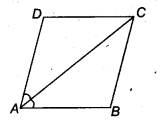Solution:
We have a parallelogram ABCD in which diagonal AC bisects ∠A
⇒ ∠DAC = ∠BAC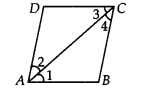(i) Since, ABCD is a parallelogram.
∴ AB || DC and AC is a transversal.
∴ ∠1 = ∠3 …(1)
[ ∵ Alternate interior angles are equal]
Also, BC || AD and AC is a transversal.
∴ ∠2 = ∠4 …(2)
[ v Alternate interior angles are equal]
Also, ∠1 = ∠2 …(3)
[ ∵ AC bisects ∠A]
From (1), (2) and (3), we have
∠3 = ∠4
⇒ AC bisects ∠C.

(ii) In ∆ABC, we have
∠1 = ∠4 [From (2) and (3)]
⇒ BC = AB …(4)
[ ∵ Sides opposite to equal angles of a ∆ are equal]
But, ABCD is a parallelogram. [Given]
∴ AB = DC …(6)
From (4), (5) and (6), we have
AB = BC = CD = DA
Thus, ABCD is a rhombus.

Question 7. ABCD is a rhombus. Show that diagonal AC bisects ∠Aas well as ∠C and diagonal BD bisects ∠B as well AS ∠D.
Solution:
Since, ABCD is a rhombus.
⇒ AB = BC = CD = DA
Also, AB || CD and AD || BC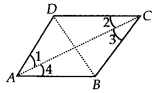Now, CD = AD ⇒ ∠1 = ∠2 …….(1)
[ ∵ Angles opposite to equal sides of a triangle are equal]
Also, AD || BC and AC is the transversal.
[ ∵ Every rhombus is a parallelogram]
⇒ ∠1 = ∠3 …(2)
[ ∵ Alternate interior angles are equal]
From (1) and (2), we have
∠2 = ∠3 …(3)
Since, AB || DC and AC is transversal.
∴ ∠2 = ∠4 …(4)
[ ∵ Alternate interior angles are equal] From (1) and (4),
we have ∠1 = ∠4
∴ AC bisects ∠C as well as ∠A.
Similarly, we can prove that BD bisects ∠B as well as ∠D.

Question 8. ABCD is a rectangle in which diagonal AC bisects ∠A as well as ∠C. Show that
(i) ABCD is a square
(ii) diagonal BD bisects ∠B as well as ∠D.

Solution:
We have a rectangle ABCD such that AC bisects ∠A as well as ∠C.
i.e., ∠1 = ∠4 and ∠2 = ∠3 ……..(1)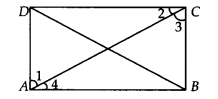(i) Since, every rectangle is a parallelogram.
∴ ABCD is a parallelogram.
⇒ AB || CD and AC is a transversal.
∴∠2 = ∠4 …(2)
[ ∵ Alternate interior angles are equal]
From (1) and (2), we have
∠3 = ∠4
In ∆ABC, ∠3 = ∠4
⇒ AB = BC
[ ∵ Sides opposite to equal angles of a A are equal]
Similarly, CD = DA
So, ABCD is a rectangle having adjacent sides equal.
⇒ ABCD is a square.

(ii) Since, ABCD is a square and diagonals of a square bisect the opposite angles.
So, BD bisects ∠B as well as ∠D.

Question 9. In parallelogram ABCD, two points P and Q are taken on diagonal BD such that DP = BQ (see figure). Show that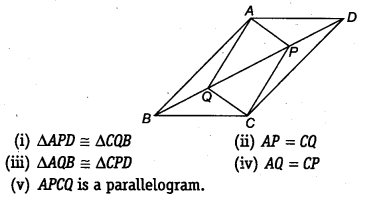Solution:
We have a parallelogram ABCD, BD is the diagonal and points P and Q are such that PD = QB

(i) Since, AD || BC and BD is a transversal.
∴ ∠ADB = ∠CBD [ ∵ Alternate interior angles are equal]
Now, in ∆APD and ∆CQB, we have
AD = CB [Opposite sides of a parallelogram ABCD are equal]
PD = QB [Given]
∴ ∆APD ≅ ∆CQB [By SAS congruency]

(ii) Since, ∆APD ≅ ∆CQB [Proved]
⇒ AP = CQ [By C.P.C.T.]

(iii) Since, AB || CD and BD is a transversal.
∴ ∠ABD = ∠CDB
⇒ ∠ABQ = ∠CDP
Now, in ∆AQB and ∆CPD, we have
QB = PD [Given]
∠ABQ = ∠CDP [Proved]
AB = CD [ Y Opposite sides of a parallelogram ABCD are equal]
∴ ∆AQB = ∆CPD [By SAS congruency]

(iv) Since, ∆AQB = ∆CPD [Proved]
⇒ AQ = CP [By C.P.C.T.]

Opposite sides are equal. [Proved]
∴ ∆PCQ is a parallelogram.

Question 10. ABCD is a parallelogram and AP and CQ are perpendiculars from vertices A and C on diagonal BD (see figure). Show that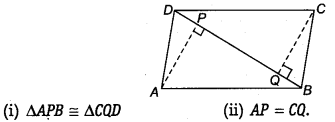Solution:
(i) In ∆APB and ∆CQD, we have
∠APB = ∠CQD [Each 90°]
AB = CD [ ∵ Opposite sides of a parallelogram ABCD are equal]
∠ABP = ∠CDQ
[ ∵ Alternate angles are equal as AB || CD and BD is a transversal]
∴ ∆APB = ∆CQD [By AAS congruency]

(ii) Since, ∆APB ≅ ∆CQD [Proved]
⇒ AP = CQ [By C.P.C.T.]

Question 11. In ∆ABC and ∆DEF, AB = DE, AB || DE, BC – EF and BC || EF. Vertices A, B and C are joined to vertices D, E and F, respectively (see figure).
Show that
(i) quadrilateral ABED is a parallelogram
(ii) quadrilateral BEFC is a parallelogram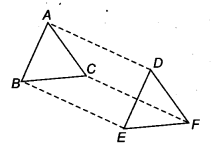(iv) quadrilateral ACFD is a parallelogram
(v) AC = DF
(vi) ∆ABC ≅ ∆DEF

Solution:
(i) We have AB = DE [Given]
and AB || DE [Given]
i. e., ABED is a quadrilateral in which a pair of opposite sides (AB and DE) are parallel and of equal length.
∴ ABED is a parallelogram.

(ii) BC = EF [Given]
and BC || EF [Given]
i.e. BEFC is a quadrilateral in which a pair of opposite sides (BC and EF) are parallel and of equal length.
∴ BEFC is a parallelogram.

(iii) ABED is a parallelogram [Proved]
[ ∵ Opposite sides of a parallelogram are equal and parallel] Also, BEFC is a parallelogram. [Proved]
BE || CF and BE = CF …(2)
[ ∵ Opposite sides of a parallelogram are equal and parallel]
From (1) and (2), we have

i.e., In quadrilateral ACFD, one pair of opposite sides (AD and CF) are parallel and of equal length.

(v) Since, ACFD is a parallelogram. [Proved]
So, AC =DF [∵ Opposite sides of a parallelogram are equal]

(vi) In ∆ABC and ∆DFF, we have
AB = DE [Given]
BC = EF [Given]
AC = DE [Proved in (v) part]
∆ABC ≅ ∆DFF [By SSS congruency]

Question 12. ABCD is a trapezium in which AB || CD and AD = BC (see figure). Show that
(i )∠A=∠B
(ii )∠C=∠D
(iv) diagonal AC = diagonal BD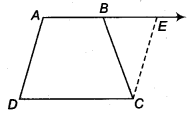[Hint: Extend AB and draw a line through C parallel to DA intersecting AB produced at E].

Solution:
We have given a trapezium ABCD in which AB || CD and AD = BC.

(i) Produce AB to E and draw CF || AD.. .(1)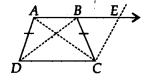∵ AB || DC
⇒ AE || DC Also AD || CF
∴ AECD is a parallelogram.
[ ∵ Opposite sides of the parallelogram are equal]
But AD = BC …(2) [Given]
By (1) and (2), BC = CF
Now, in ∆BCF, we have BC = CF
⇒ ∠CEB = ∠CBE …(3)
[∵ Angles opposite to equal sides of a triangle are equal]
Also, ∠ABC + ∠CBE = 180° … (4)
[Linear pair]
and ∠A + ∠CEB = 180° …(5)
[Co-interior angles of a parallelogram ADCE]
From (4) and (5), we get
∠ABC + ∠CBE = ∠A + ∠CEB
⇒ ∠ABC = ∠A [From (3)]
⇒ ∠B = ∠A …(6)

(ii) AB || CD and AD is a transversal.
∴ ∠A + ∠D = 180° …(7) [Co-interior angles]
Similarly, ∠B + ∠C = 180° … (8)
From (7) and (8), we get
∠A + ∠D = ∠B + ∠C
⇒ ∠C = ∠D [From (6)]

(iii) In ∆ABC and ∆BAD, we have
AB = BA [Common]
∴ ∆ABC = ∆BAD [By SAS congruency]

(iv) Since, ∆ABC = ∆BAD [Proved]
⇒ AC = BD [By C.P.C.T.]

### NCERT Solutions for Class 9 Maths Exercise 8.2

Question 1. ABCD is a quadrilateral in which P, Q, R and S are the mid-points of sides AB, BC, CD and DA respectively (See figure). AC is a diagonal. Show that :

(i) SR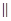AC and SR =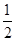AC

(ii) PQ = SR

(iii) PQRS is a parallelogram.

Solution:
In ABC, P is the mid-point of AB and Q is the mid-point of BC.

Then PQAC and PQ =AC

(i) In ACD, R is the mid-point of CD and S is the mid-point of AD.

Then SRAC and SR =AC

(ii) Since PQ =AC and SR =AC

Therefore, PQ = SR

(iii) Since PQAC and SRAC

Therefore, PQSR [two lines parallel to given line are parallel to each other]

Now PQ = SR and PQSR

Therefore, PQRS is a parallelogram.

Question 2. ABCD is a rhombus and P, Q, R, S are mid-points of AB, BC, CD and DA respectively. Prove that quadrilateral PQRS is a rectangle.

Solution:
Given: P, Q, R and S are the mid-points of respective sides AB, BC, CD and DA of rhombus. PQ, QR, RS and SP are joined.

To prove: PQRS is a rectangle.

Construction: Join A and C.

Proof: In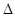ABC, P is the mid-point of AB and Q is the mid-point of BC.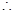PQAC and PQ =AC ……….(i)

InADC, R is the mid-point of CD and S is the mid-point of AD.SRAC and SR =AC ……….(ii)

From eq. (i) and (ii), PQSR and PQ = SRPQRS is a parallelogram.

Now ABCD is a rhombus. [Given]AB = BC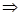AB =BCPB = BQ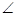1 =2 [Angles opposite to equal sides are equal]

Now in triangles APS and CQR, we have,

AP = CQ [P and Q are the mid-points of AB and BC and AB = BC]

Similarly, AS = CR and PS = QR [Opposite sides of a parallelogram]APS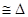CQR [By SSS congreuancy]3 =4 [By C.P.C.T.]

Now we have1 +SPQ +3 =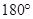And2 +PQR +4 =[Linear pairs]1 +SPQ +3 =2 +PQR +4

Since1 =2 and3 =4 [Proved above]SPQ =PQR ……….(iii)

Now PQRS is a parallelogram [Proved above]SPQ +PQR =……….(iv) [Interior angles]

Using eq. (iii) and (iv),SPQ +SPQ =2SPQ =SPQ =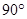Hence PQRS is a rectangle.

Question 3. ABCD is a rectangle and P, Q, R and S are the mid-points of the sides AB, BC, CD and DA respectively. Show that the quadrilateral PQRS is a rhombus.

Solution:
Given: A rectangle ABCD in which P, Q, R and S are the mid-points of the sides AB, BC, CD and DA respectively. PQ, QR, RS and SP are joined.

To prove: PQRS is a rhombus.

Construction: Join AC.

Proof: In ABC, P and Q are the mid-points of sides AB, BC respectively.

PQ AC and PQ = AC ……….(i)

In ADC, R and S are the mid-points of sides CD, AD respectively.

SR AC and SR = AC ……….(ii)

From eq. (i) and (ii), PQ SR and PQ = SR ……….(iii)

PQRS is a parallelogram.

Now ABCD is a rectangle. [Given]

AD = BC AS = BQ ……….(iv)

In triangles APS and BPQ,

AP = BP [P is the mid-point of AB]

PAS = PBQ [Each ]

And AS = BQ [From eq. (iv)]

APS BPQ [By SAS congruency]

PS = PQ [By C.P.C.T.] ………(v)

From eq. (iii) and (v), we get that PQRS is a parallelogram.

PS = PQ

Hence, PQRS is a rhombus.

Question 4. ABCD is a trapezium, in which AB DC, BD is a diagonal and E is the mid-point of AD. A line is drawn through E, parallel to AB intersecting BC at F (See figure). Show that F is the mid-point of BC.

Solution:
Let diagonal BD intersect line EF at point P.

In DAB,

E is the mid-point of AD and EP AB [ EF AB (given) P is the part of EF]

P is the mid-point of other side, BD of DAB.

[A line drawn through the mid-point of one side of a triangle, parallel to another side intersects the third side at the mid-point]

Now in BCD,

P is the mid-point of BD and PF DC [EF AB (given) and AB DC (given)]

EF DC and PF is a part of EF.

F is the mid-point of other side, BC of BCD. [Converse of mid-point of theorem]

Question 5. In a parallelogram ABCD, E and F are the mid-points of sides AB and CD respectively (See figure). Show that the line segments AF and EC trisect the diagonal BD.

Solution:
Since E and F are the mid-points of AB and CD respectively.

AE = AB and CF = CD……….(i)

But ABCD is a parallelogram.

AB = CD and AB DC

AB = CD and AB DC

AE = FC and AE FC [From eq. (i)]

AECF is a parallelogram.

FA CE FP CQ [FP is a part of FA and CQ is a part of CE] ………(ii)

Since the segment drawn through the mid-point of one side of a triangle and parallel to the other side bisects the third side.

In DCQ, F is the mid-point of CD and FP CQ

P is the mid-point of DQ.

DP = PQ ……….(iii)

Similarly, In ABP, E is the mid-point of AB and EQ AP

Q is the mid-point of BP.

BQ = PQ ……….(iv)

From eq. (iii) and (iv),

DP = PQ = BQ ………(v)

Now BD = BQ + PQ + DP = BQ + BQ + BQ = 3BQ

BQ = BD ……….(vi)

From eq. (v) and (vi),

DP = PQ = BQ = BD

Points P and Q trisects BD.

So AF and CE trisects BD.

Question 6. Show that the line segments joining the mid-points of opposite sides of a quadrilateral bisect each other.

Solution:
Given: A quadrilateral ABCD in which EG and FH are the line-segments joining the mid-points of opposite sides of a quadrilateral.

To prove: EG and FH bisect each other.

Construction: Join AC, EF, FG, GH and HE.

Proof: In ABC, E and F are the mid-points of respective sides AB and BC.

EF AC and EF AC ……….(i)

G and H are the mid-points of respective sides CD and AD.

HG AC and HG AC ……….(ii)

From eq. (i) and (ii),

EF HG and EF = HG

EFGH is a parallelogram.

Since the diagonals of a parallelogram bisect each other, therefore line segments (i.e. diagonals) EG and FH (of parallelogram EFGH) bisect each other.

Question 7. ABC is a triangle right angled at C. A line through the mid-point M of hypotenuse AB and parallel to BC intersects AC at D.

Solution:
(i) In ABC, M is the mid-point of AB [Given]

MD BC

AD = DC [Converse of mid-point theorem]

Thus D is the mid-point of AC.

(ii) BC (given) consider AC as a transversal.

1 = C [Corresponding angles]

1 = [C = ]

Thus MD AC.

(iii) In AMD and CMD,

1 = 2 = [proved above]

MD = MD [common]

AMD CMD [By SAS congruency]

AM = CM [By C.P.C.T.] ……….(i)

Given that M is the mid-point of AB.

AM = AB ……….(ii)

From eq. (i) and (ii),

CM = AM = AB

## Conclusions for NCERT SOLUTIONS FOR CLASS 9 MATHS CHAPTER 8 QUADRILATERALS

SWC academic staff has developed NCERT answers for this chapter of the ninth grade mathematics curriculum. We have solutions prepared for all of the exercises of this chapter. The answers, broken down into steps, to all of the questions included in the NCERT textbook’s chapter are provided here. Read this chapter on theory. Be certain that you have read the theory section of this chapter of the NCERT textbook and that you have learnt the formulas for the chapter that you are studying.